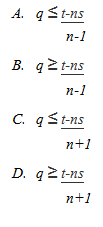# Aptitude | GATE CS 1998 | Question 52

Consider n processes sharing the CPU in a round-robin fashion. Assuming that each process switch takes s seconds, what must be the quantum size q such that the overhead resulting from process switching is minimized but, at the same time, each process is guaranteed to get its turn at the CPU at least every t seconds ?(A) a
(B) b
(C) c
(D) d

Explanation: Each process will get CPU for q seconds and each process wants CPU again after t seconds.
Thus, there will be (n-1) processes once after current process gets CPU again. Each process takes s seconds for context switch.

`(Qp1)(s)(Qp2)(s)(Qp3)(s)(Qp1) `

It can be seen that since P1 left and arrived again, there have been n context switches and (n-1) processes. Thus, equation will be:

```q*(n-1) + n*s <= t
q*(n-1) <= t - n*s
q <= (t-n.s) / (n-1) ```

So, option (A) is correct.

Quiz of this Question

My Personal Notes arrow_drop_up

Improved By : parthendo

Article Tags :

1

Please write to us at contribute@geeksforgeeks.org to report any issue with the above content.